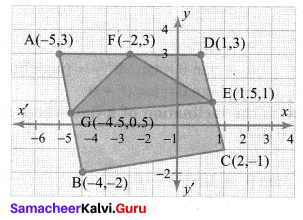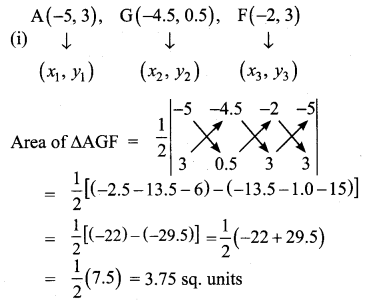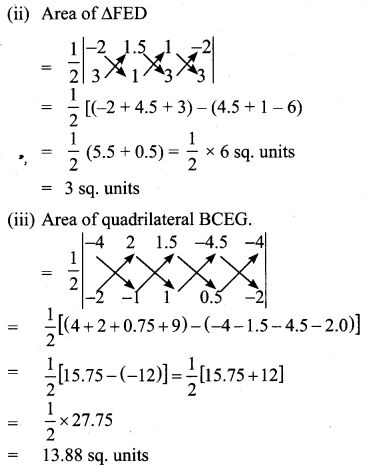# Samacheer Kalvi 10th Maths Solutions Chapter 5 Coordinate Geometry Ex 5.1

## Tamilnadu Samacheer Kalvi 10th Maths Solutions Chapter 5 Coordinate Geometry Ex 5.1

10th Maths Exercise 5.1 Samacheer Kalvi Question 1.
Find the area of the triangle formed by the points
(i) (1, -1), (-4, 6) and (-3, -5)
(ii) (-10, -4), (-8, -1) and (-3, -5)
Solution: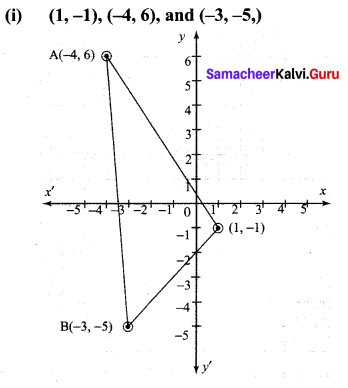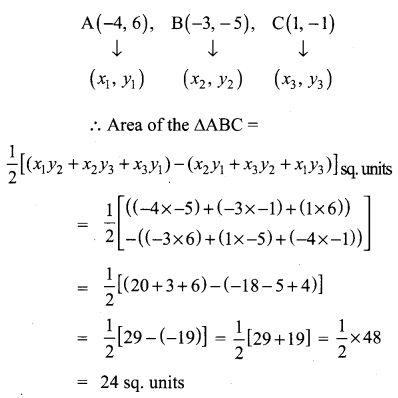(ii) (-10, -4), (-8, -1) and (-3, -5)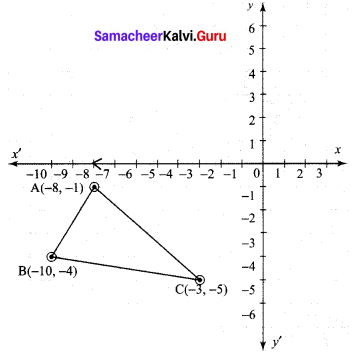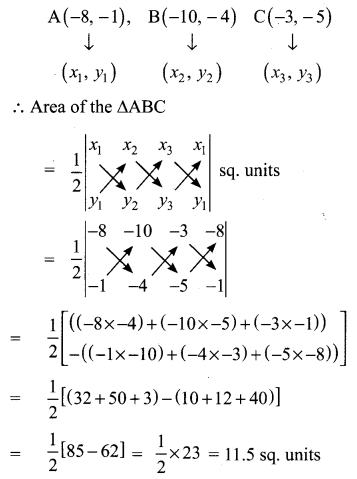Exercise 5.1 Class 10 Samacheer Kalvi Question 2.
Detemine whether the sets of points are collinear ?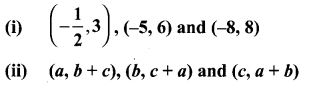Solution:
(i)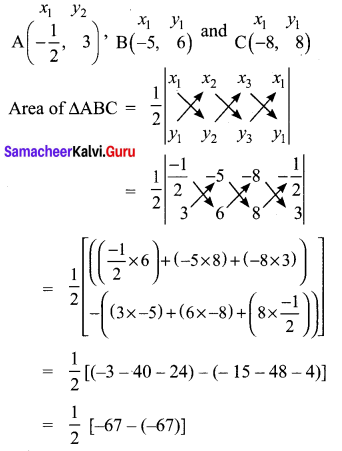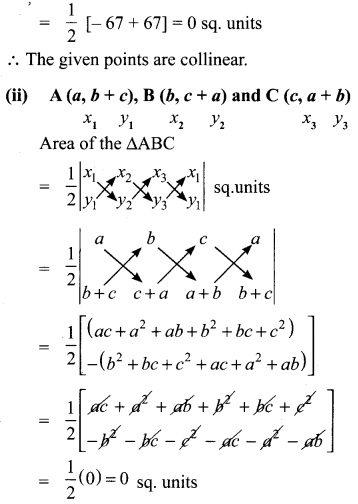∴ The given points are collinear

Ex 5.1 Class 10 Samacheer Question 3.
Vertices of given triangles are taken in order and their areas are provided aside. In each case, find the value of ‘p’.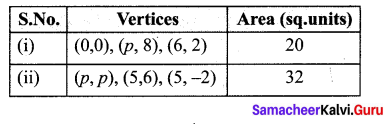Solution:
Area 20 sq. units.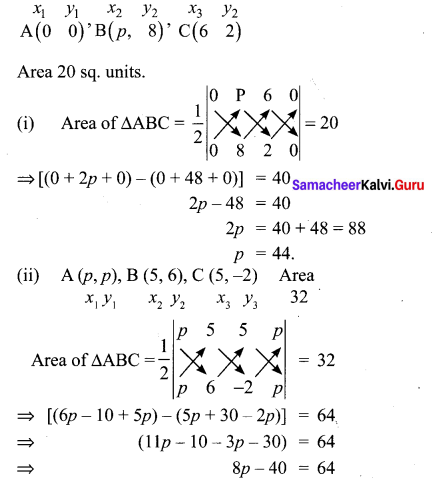8p = 104
p = 13

10th Maths Exercise 5.1 Question 4.
In each of the following, find the value of ‘a’ for which the given points are collinear.
(i) (2, 3), (4, a) and (6, -3)
(ii) (a, 2 – 2a), (-a + 1, 2a) and(-4 – a,6 – 2a)
Solution: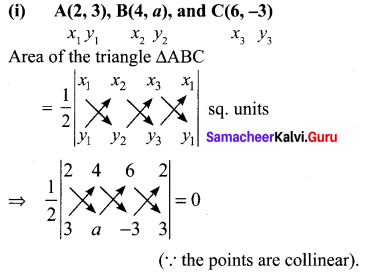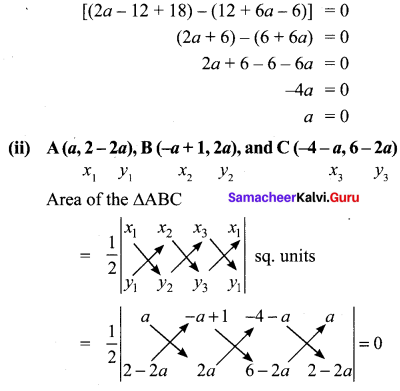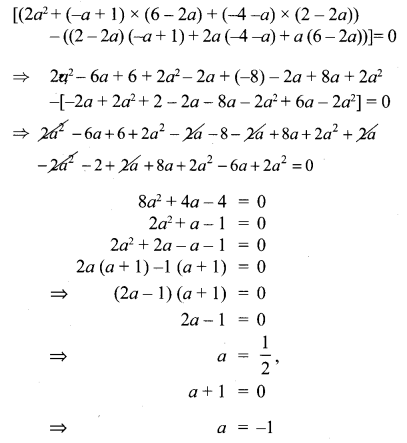10th Maths Coordinate Geometry Exercise 5.1 Question 5.
Find the area of the quadrilateral whose vertices are at
(i) (-9, -2), (-8, -4), (2, 2) and (1, -3)
(ii) (-9, 0), (-8, 6), (-1, -2) and (-6, -3)
Solution:
(i) (-9, -2), (-8, -4), (2, 2), and (1, -3)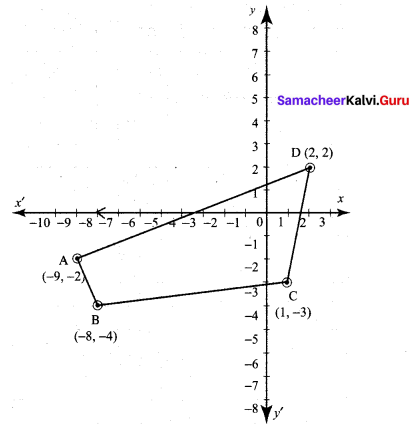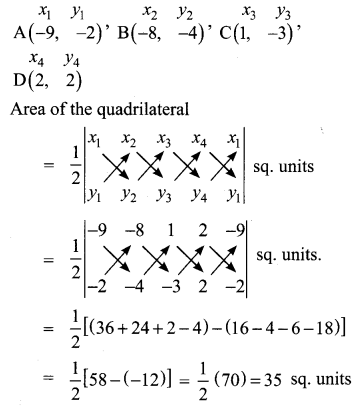(ii) (-9, 0), (-8, 6), (-1, -2) and (-6, -3)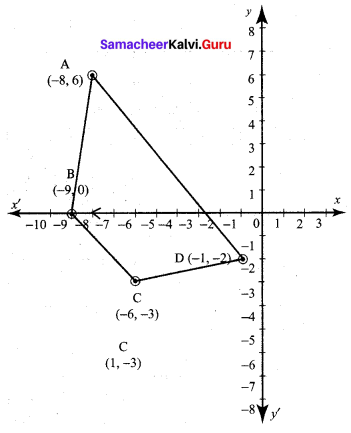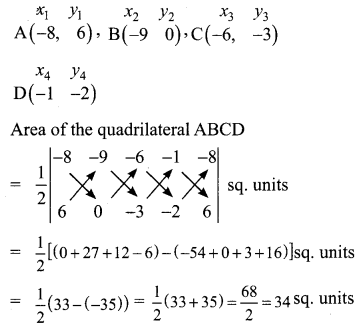10th Maths Ex 5.1 Question 6.
Find the value of k, if the area ofa quadrilateral is 28 sq.units, whose vertices are (-4, -2), (-3, k), (3, -2) and (2, 3)
Solution: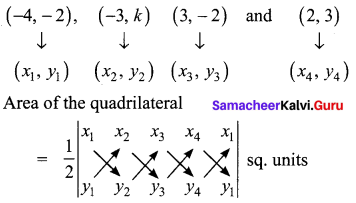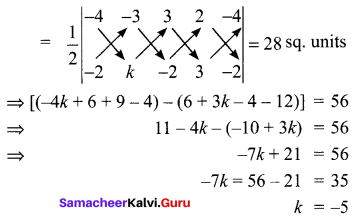10th Maths 5.1 Question 7.
If the points A(-3, 9) , B(a, b) and C(4,-5) are collinear and if a + b = 1, then find a and b.
Solution: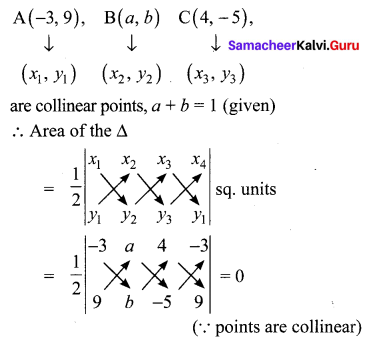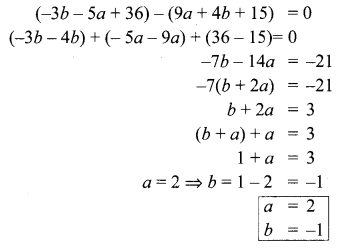10th Standard Maths Exercise 5.1 Question 8.
Let P(11, 7), Q(13.5, 4) and R(9.5, 4) be the mid-points of the sides AB, BC and AC respectively of ∆ABC . Find the coordinates of the vertices A, B and C. Hence find the area of ∆ABC and compare this with area of ∆PQR.
Solution:
p (11, 7), Q (13.5, 4), and R (9.5, 4) are the mid points of the sides of a ∆ABC.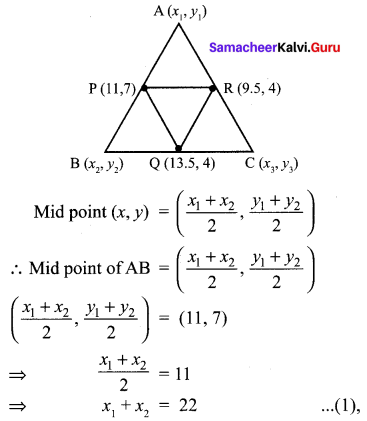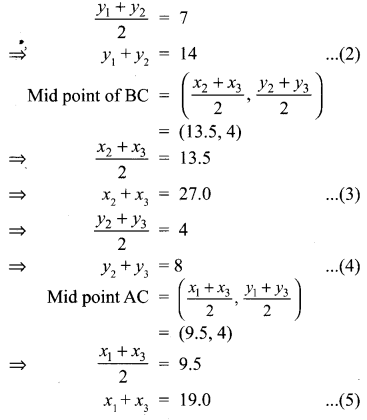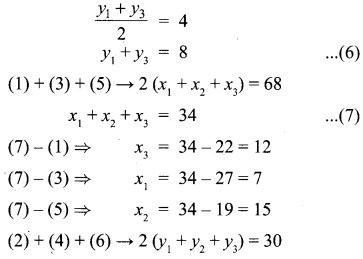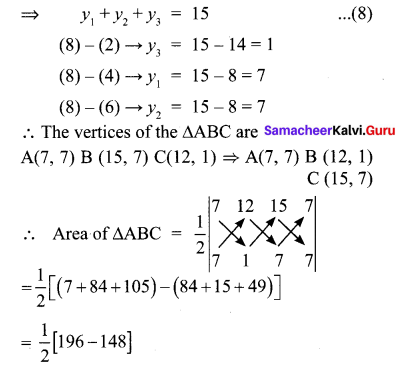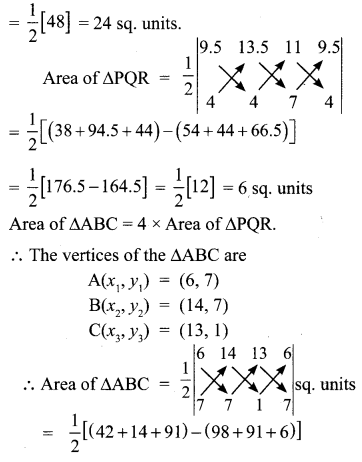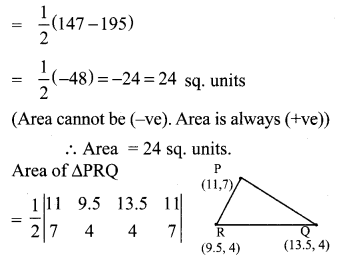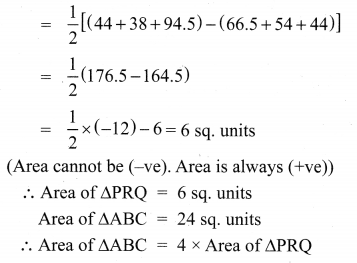Maths Ex 5.1 Class 10 Samacheer Question 9.
In the figure, the quadrilateral swimming pool shown is surrounded by concrete patio. Find the area of the patio.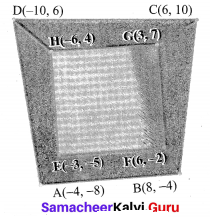Solution:
Area of the patio = Area of the quadrilateral ABCD – Area of the swimming pool EFGFI.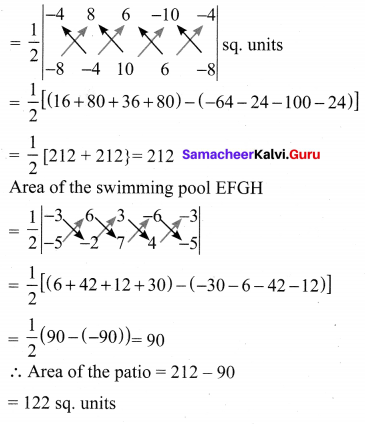10th Coordinate Geometry 5.1 Question 10.
A triangular shaped glass with vertices at A(-5, -4), B(1, 6) and C(7, -4) has to be painted.
If one bucket of paint covers 6 square feet, how many buckets of paint will be required to paint the whole glass, if only one coat of paint is applied.
Solution: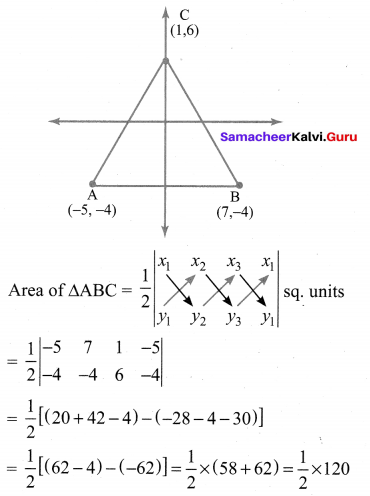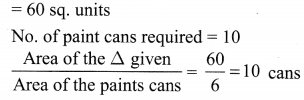10th Samacheer Kalvi Maths Coordinate Geometry Question 11.
In the figure, find the area of
(i) triangle AGF
(ii) triangle FED